# 【python小课堂专栏】python小课堂38 - 关于 *args,**kwargs 的作用

## python小课堂38 - 关于 *args,**kwargs 的作用

### *args 用法

*args：可以理解为多个无名参数，也有人叫可变位置参数的。

def print_func(*args):
print(type(args))
print(args)

print_func(1, 2, '呵呵哒', [])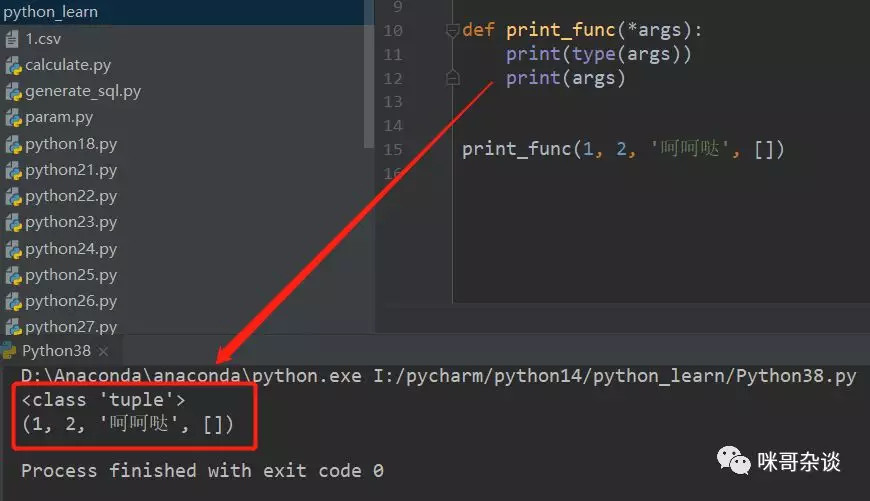def print_func(x, y, *args):
print(type(x))
print(x)
print(y)
print(type(args))
print(args)

print_func(1, 2, '呵呵哒', [])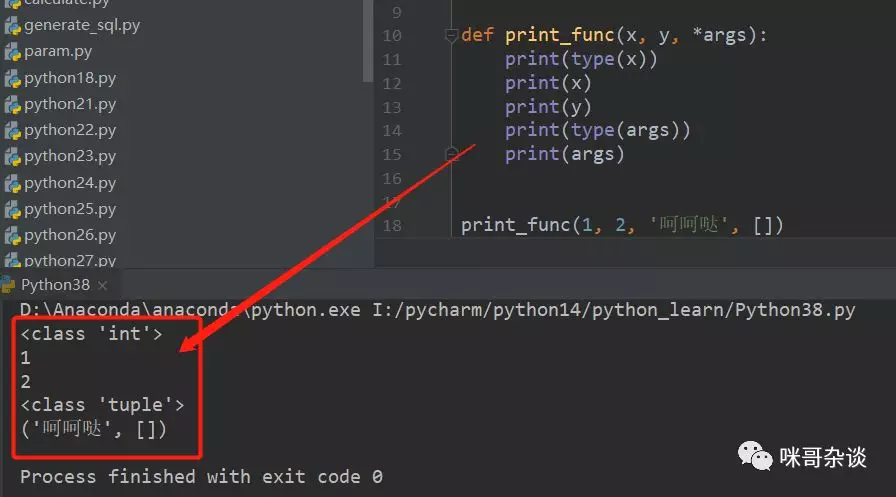def print_func(*args, x, y):
print(type(x))
print(x)
print(y)
print(type(args))
print(args)

print_func(1, 2, '呵呵哒', [])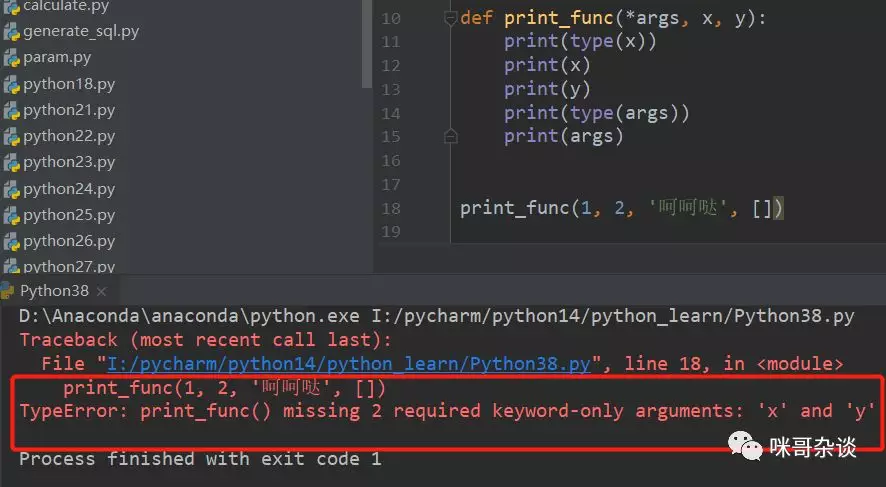def print_func(*args, x, y):
print(type(x))
print(x)
print(y)
print(type(args))
print(args)

print_func(1, 2, '呵呵哒', [], x='x', y='y')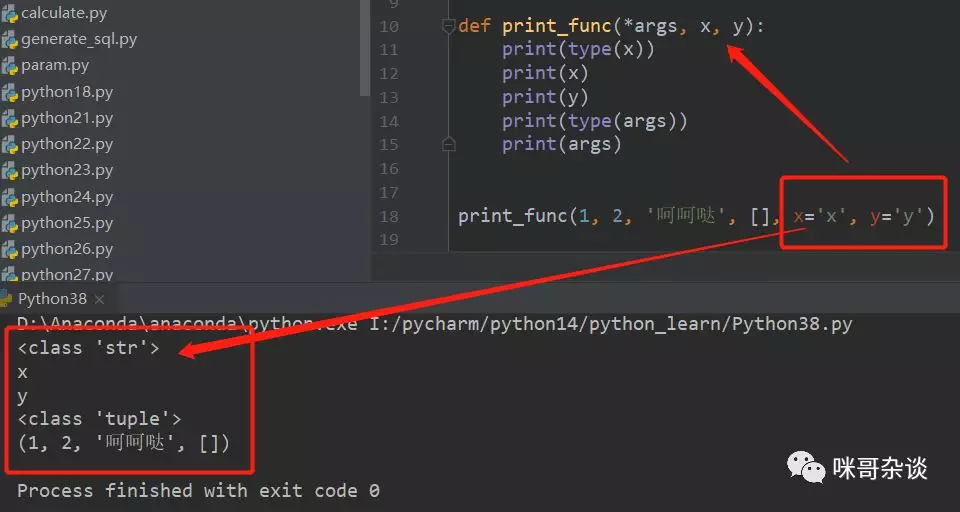1. (x,y,*args) 顺序

2. (*args,x,y) 顺序

### **kwargs 用法

**kwargs：多个关键词参数。


def print_func(**kwargs):
print(type(kwargs))
print(kwargs)

print_func(1, 2, '呵呵哒', [])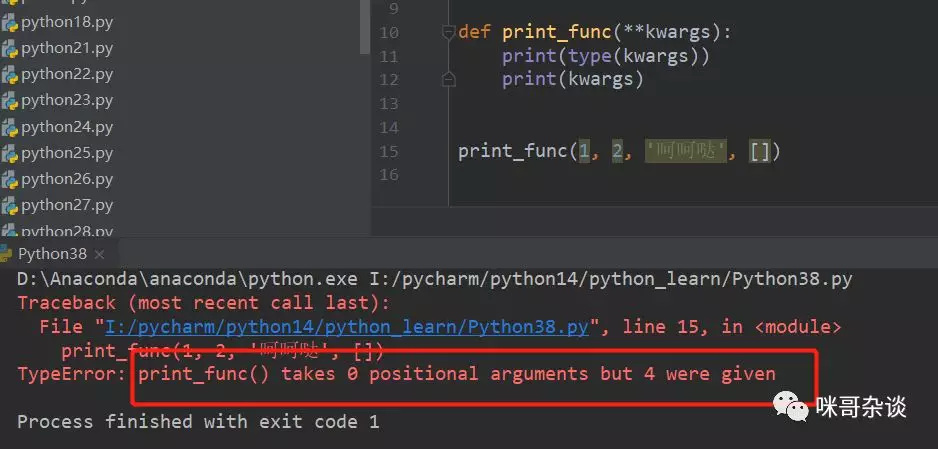def print_func(**kwargs):
print(type(kwargs))
print(kwargs)

print_func(a=1, b=2, c='呵呵哒', d=[])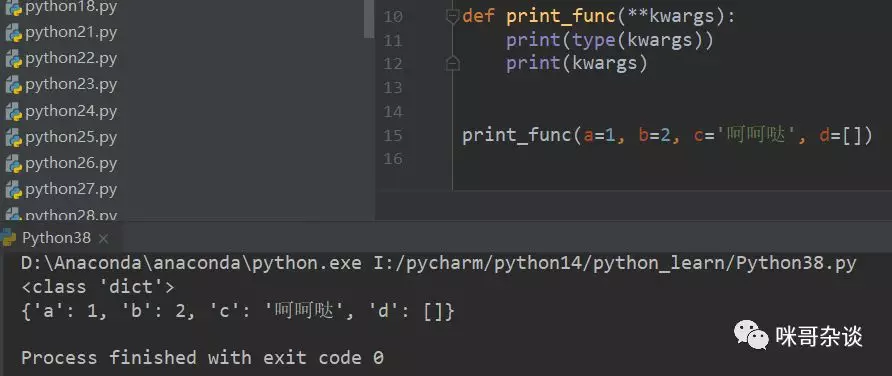### 组合使用

arg,*args,**kwargs,三者是可以组合使用的，但是组合使用需要遵循一定的语法规则，即顺序为王。

arg,*args,**kwargs作为函数参数的顺序。

def print_func(x, *args, **kwargs):
print(x)
print(args)
print(kwargs)

print_func(1, 2, 3, 4, y=1, a=2, b=3, c=4)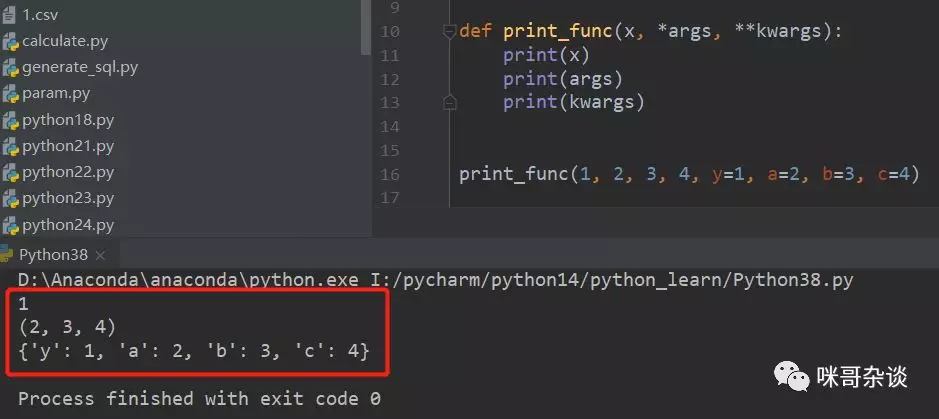### 你不知道的小知识点

def print_func(a, b, c):
print(f'a = {a}, b = {b}, c = {c}')

animal_list = ['pig', 'dog', 'cat']
print_func(*animal_list)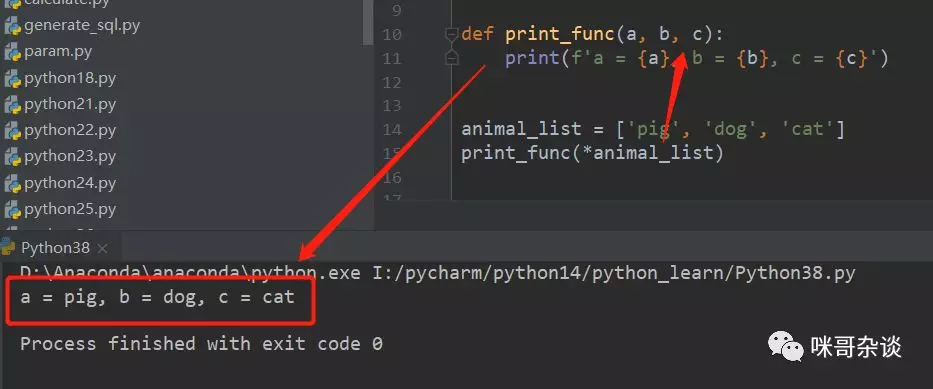def print_func(a, b, c):
print(f'a : {a}, b : {b}, c : {c}')

animal_dict = {'a': 'pig', 'b': 'dog', 'c': 'cat'}
print_func(**animal_dict)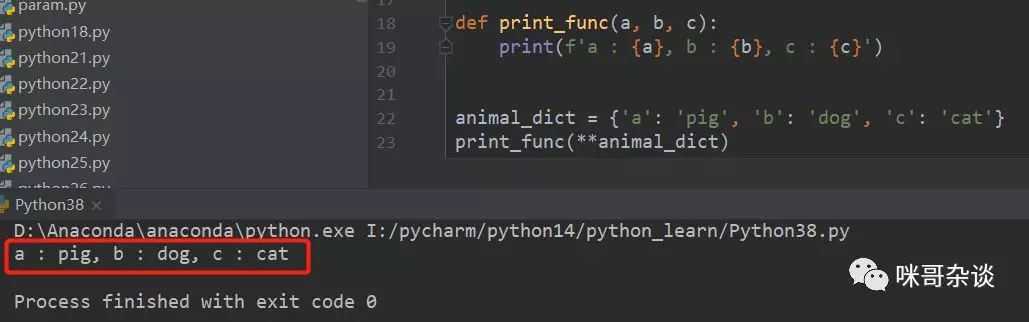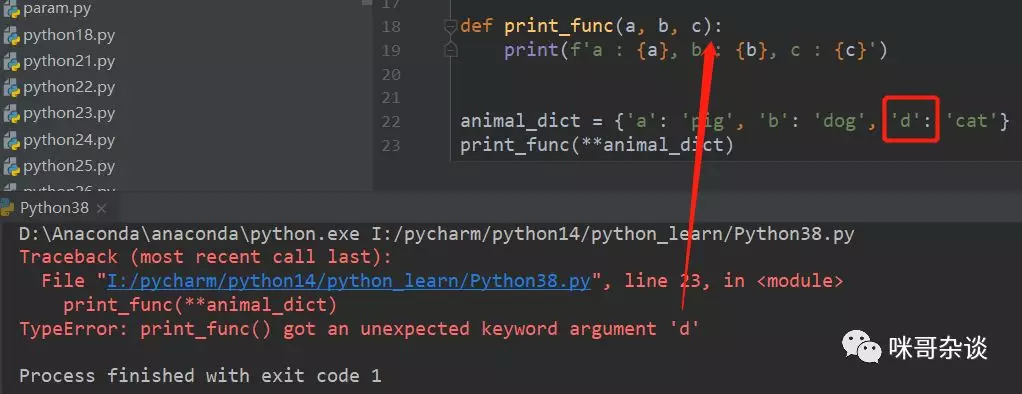### 总结

11-203702-18108
01-093万+
07-213944
11-104万+
10-22565
12-126006
06-061604
05-031093
08-221万+
05-24610
04-024847
02-28222
10-09559
01-1822
05-121806
09-261593
04-2431
05-23188